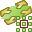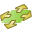# Infinite Planes and Half-Spaces

Use an infinite plane or half-space to model a ground plane efficiently. The number of triangles in the model is reduced as the ground plane is not discretised into triangles.

On the Construct tab, in the Structures group, click thePlanes/Arrays icon. From the drop-down list, selectPlane / Ground.

## No Ground (Homogeneous Free Space Medium) [Default]

The model is solved in a homogeneous environment filled with free space medium. Edit the properties of free space if required.

## Perfect Electric (PEC) Ground Plane at Z=0

Add an infinite PEC ground plane at Z=0 (in the global coordinate system) using the exact reflection coefficients, where the reflected field is added to get the total field.
Note: For a PEC ground plane, dielectric and metallic faces may connect to the ground plane and may coincide with ground plane, but may not cross or be below the ground plane.

## Perfect Magnetic (PMC) Ground Plane at Z=0

Add an infinite PMC ground plane at Z=0 (in the global coordinate system) using the exact reflection coefficients, where the reflected field is added to get the total field.
Note: For a PMC ground plane, only metallic faces may connect to the ground plane, but may not coincide with the ground plane.

## Homogeneous Half Space in Region Z<0 (Reflection Coefficient Approximation)

Add an infinite dielectric or a metallic half space for Z<0 with the boundary at Z=0 (in the global coordinate system). The half space uses the reflection coefficient ground plane approximation, where the reflected field is added to get the total field.
Note:
• For the reflection coefficient approximation ground, the structures must be above (Z>0) and at least $\frac{\lambda }{10}$ away from the ground plane, where $\lambda$ is the free space wavelength.
• This technique is faster and potentially less accurate than the exact Sommerfeld integrals method.

## Homogeneous Half Space in Region Z<0 (Exact Sommerfeld Integrals)

Add an infinite dielectric ground plane for Z<0 with the boundary at Z=0 (in the global coordinate system). The half space uses the Sommerfeld integrals to solve the exact boundary condition with the appropriate Green's function.
Note:
• A dielectric face may not coincide with the Z=0 half-space boundary.
• A metallic face may coincide with the Z=0 half-space boundary.
• Structures may cross the Z=0 half-space boundary.
• Structures may be inside (Z<0) the half-space boundary.

## Planar Multilayer Substrate

Add a planar multilayer substrate (finite or infinite) orthogonal to the Z axis (in the global coordinate system).
Note:
• Supports arbitrarily shaped structures inside the substrate. Structures may cross multiple layers.
• Enclose the substrate in a MoM / SEP region to create a finite planar multilayer substrate.